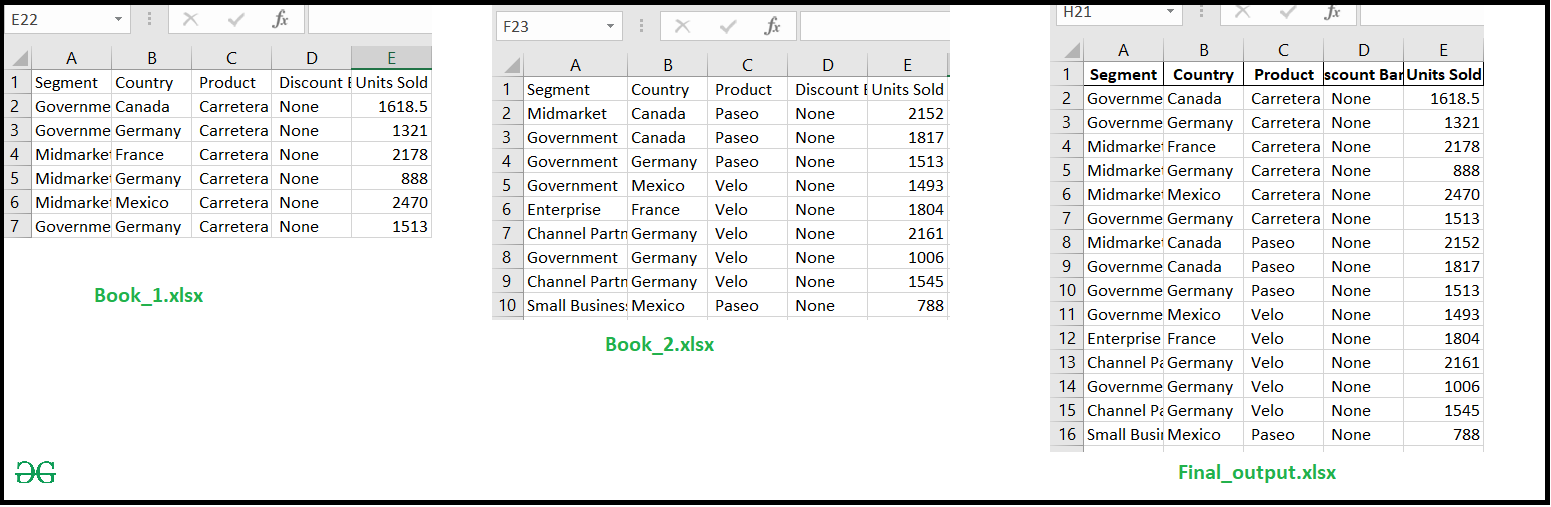Combine Multiple Excel Worksheets Into a Single Pandas Dataframe

• Last Updated : 05 Sep, 2020

Prerequisites: Working with excel files using Pandas

In these articles, we will discuss how to Import multiple excel sheet into a single DataFrame and save into a new excel file. Let’s suppose we have two Excel files with the same structure (Excel_1.xlsx, Excel_2.xlsx), then merge both of the sheets into a new Excel file.

Approach :

• Import-Module
• Read Excel file and store into a DataFrame
• Concat both DataFrame into a new DataFrame
• Export DataFrame into an Excel File with DataFrame.to_excel() function

Below is the implementation.

Python3

 # import moduleimport pandas as pd  # Read excel file# and store into a DataFramedf1 = pd.read_excel('excel_work\sample_data\Book_1.xlsx')df2 = pd.read_excel('excel_work\sample_data\Book_2.xlsx')  # concat both DataFrame into a single DataFramedf = pd.concat([df1, df2])  # Export Dataframe into Excel filedf.to_excel('final_output.xlsx', index=False)

Output :Attention geek! Strengthen your foundations with the Python Programming Foundation Course and learn the basics.

To begin with, your interview preparations Enhance your Data Structures concepts with the Python DS Course. And to begin with your Machine Learning Journey, join the Machine Learning - Basic Level Course

My Personal Notes arrow_drop_up